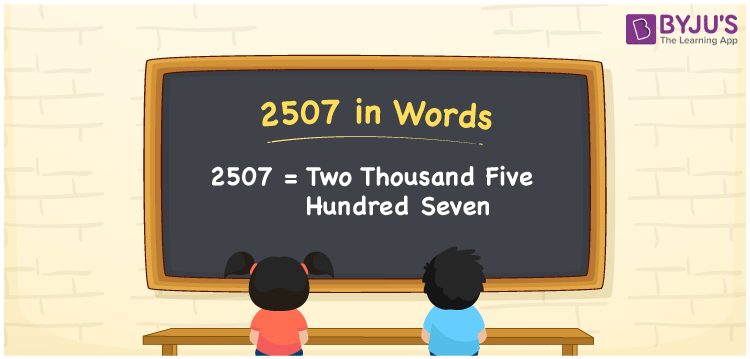2507 in words is written as Two Thousand Five Hundred and Seven. 2507 represents the count or value. The article on Counting Numbers can give you an idea about count or counting. The number 2507 is used in expressions that relate to money, distance, length, year and others. Let us consider an example for 2507. ”Can I buy a bicycle with Two Thousand Five Hundred and Seven rupees?”

 2507 in words Two Thousand Five Hundred and Seven Two Thousand Five Hundred and Seven in Numbers 2507

## 2507 in English Words## How to Write 2507 in Words?

We can convert 2507 to words using a place value chart. The number 2507 has 4 digits, so let’s make a chart that shows the place value up to 4 digits.

 Thousands Hundreds Tens Ones 2 5 0 7

Thus, we can write the expanded form as:

2 × Thousand + 5 × Hundred + 0 × Ten + 7 × One

= 2 × 1000 + 5 × 100 + 0 × 10 + 7 × 1

= 2507

= Two Thousand Five Hundred and Seven.

2507 is the natural number that is succeeded by 2506 and preceded by 2508.

2507 in words – Two Thousand Five Hundred and Seven.

Is 2507 an odd number? – Yes.

Is 2507 an even number? – No.

Is 2507 a perfect square number? – No.

Is 2507 a perfect cube number? – No.

Is 2507 a prime number? – No.

Is 2507 a composite number? – Yes.

## Solved Example

1. Write the number 2507 in expanded form

Solution: 2 × 1000 + 5 × 100 + 0 × 10 + 7 × 1

We can write 2507 = 2000 + 500 + 00 + 7

= 2 × 1000 + 5 × 100 + 0 × 10 + 7 × 1

## Frequently Asked Questions on 2507 in words

Q1

### How to write 2507 in words?

2507 in words is written as Two Thousand Five Hundred and Seven.
Q2

### Is 2507 a perfect square number?

No. 2507 is not a perfect square number.
Q3

### Is 2507 a prime number?

No. 2507 is not a prime number.